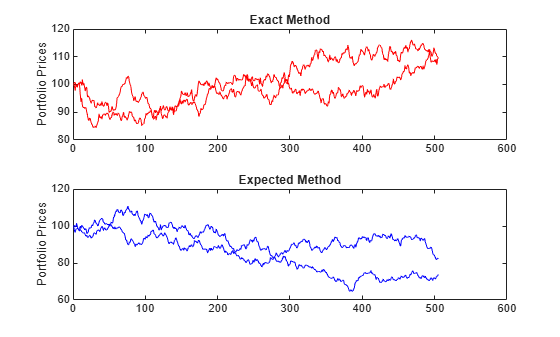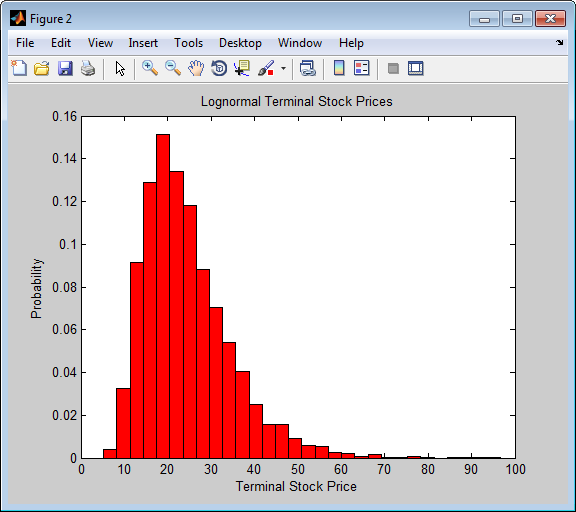Documentation

# portsim

Monte Carlo simulation of correlated asset returns

## Syntax

``RetSeries = portsim(ExpReturn,ExpCovariance,NumObs)``
``RetSeries = portsim(___,RetIntervals,NumSim,Method)``

## Description

example

````RetSeries = portsim(ExpReturn,ExpCovariance,NumObs)` simulates correlated returns of `NASSETS` assets over `NUMOBS` consecutive observation intervals. Asset returns are simulated as the proportional increments of constant drift, constant volatility stochastic processes, thereby approximating continuous-time geometric Brownian motion.```

example

````RetSeries = portsim(___,RetIntervals,NumSim,Method)` specifies options using one or more optional arguments in addition to the input arguments in the previous syntax. ```

## Examples

collapse all

This example shows the distinction between the `Exact` and `Expected` methods of simulation.

Consider a portfolio of five assets with the following expected returns, standard deviations, and correlation matrix based on daily asset returns (where `ExpReturn` and `Sigmas` are divided by 100 to convert percentages to returns).

```ExpReturn = [0.0246 0.0189 0.0273 0.0141 0.0311]/100; Sigmas = [0.9509 1.4259 1.5227 1.1062 1.0877]/100; Correlations = [1.0000 0.4403 0.4735 0.4334 0.6855 0.4403 1.0000 0.7597 0.7809 0.4343 0.4735 0.7597 1.0000 0.6978 0.4926 0.4334 0.7809 0.6978 1.0000 0.4289 0.6855 0.4343 0.4926 0.4289 1.0000];```

Convert the correlations and standard deviations to a covariance matrix.

`ExpCovariance = corr2cov(Sigmas, Correlations)`
```ExpCovariance = 5×5 10-3 × 0.0904 0.0597 0.0686 0.0456 0.0709 0.0597 0.2033 0.1649 0.1232 0.0674 0.0686 0.1649 0.2319 0.1175 0.0816 0.0456 0.1232 0.1175 0.1224 0.0516 0.0709 0.0674 0.0816 0.0516 0.1183 ```

Assume that there are 252 trading days in a calendar year, and simulate two sample paths (realizations) of daily returns over a two-year period. Since `ExpReturn` and `ExpCovariance` are expressed daily, set `RetIntervals = 1`.

```StartPrice = 100; NumObs = 504; % two calendar years of daily returns NumSim = 2; RetIntervals = 1; % one trading day NumAssets = 5;```

To illustrate the distinction between methods, simulate two paths by each method, starting with the same random number state.

```rng('default'); RetExact = portsim(ExpReturn, ExpCovariance, NumObs, ... RetIntervals, NumSim, 'Exact'); rng(0); RetExpected = portsim(ExpReturn, ExpCovariance, NumObs, ... RetIntervals, NumSim, 'Expected');```

Compare the mean and covariance of `RetExact` with the inputs (`ExpReturn` and `ExpCovariance`), you will observe that they are almost identical.

At this point, `RetExact` and `RetExpected` are both 504-by-5-by-2 arrays. Now assume an equally weighted portfolio formed from the five assets and create arrays of portfolio returns in which each column represents the portfolio return of the corresponding sample path of the simulated returns of the five assets. The portfolio arrays `PortRetExact` and `PortRetExpected` are 504-by-2 matrices.

```Weights = ones(NumAssets, 1)/NumAssets; PortRetExact = zeros(NumObs, NumSim); PortRetExpected = zeros(NumObs, NumSim); for i = 1:NumSim PortRetExact(:,i) = RetExact(:,:,i) * Weights; PortRetExpected(:,i) = RetExpected(:,:,i) * Weights; end```

Finally, convert the simulated portfolio returns to prices and plot the data. In particular, note that since the `Exact` method matches expected return and covariance, the terminal portfolio prices are virtually identical for each sample path. This is not true for the `Expected` simulation method. Although this example examines portfolios, the same methods apply to individual assets as well. Thus, `Exact` simulation is most appropriate when unique paths are required to reach the same terminal prices.

```PortExact = ret2tick(PortRetExact, ... repmat(StartPrice,1,NumSim)); ```
```Warning: This syntax will be removed in a future release. See the documentation for recommended usage. ```
```PortExpected = ret2tick(PortRetExpected, ... repmat(StartPrice,1,NumSim)); ```
```Warning: This syntax will be removed in a future release. See the documentation for recommended usage. ```
```subplot(2,1,1), plot(PortExact, '-r') ylabel('Portfolio Prices') title('Exact Method') subplot(2,1,2), plot(PortExpected, '-b') ylabel('Portfolio Prices') title('Expected Method')```This example shows the interplay among `ExpReturn`, `ExpCovariance`, and `RetIntervals`. Recall that `portsim` simulates correlated asset returns over an interval of length dt, given by the equation

`$\frac{dS}{S}=\mu dt+\sigma dz=\mu dt+\sigma \epsilon \sqrt{dt},$`

where S is the asset price, μ is the expected rate of return, σ is the volatility of the asset price, and ε represents a random drawing from a standardized normal distribution.

The time increment dt is determined by the optional input `RetIntervals`, either as an explicit input argument or as a unit time increment by default. Regardless, the periodicity of `ExpReturn`, `ExpCovariance`, and `RetIntervals` must be consistent. For example, if `ExpReturn` and `ExpCovariance` are annualized, then `RetIntervals` must be in years. This point is often misunderstood.

To illustrate the interplay among `ExpReturn`, `ExpCovariance`, and `RetIntervals`, consider a portfolio of five assets with the following expected returns, standard deviations, and correlation matrix based on daily asset returns.

```ExpReturn = [0.0246 0.0189 0.0273 0.0141 0.0311]/100; Sigmas = [0.9509 1.4259 1.5227 1.1062 1.0877]/100; Correlations = [1.0000 0.4403 0.4735 0.4334 0.6855 0.4403 1.0000 0.7597 0.7809 0.4343 0.4735 0.7597 1.0000 0.6978 0.4926 0.4334 0.7809 0.6978 1.0000 0.4289 0.6855 0.4343 0.4926 0.4289 1.0000]; ```

Convert the correlations and standard deviations to a covariance matrix of daily returns.

``` ExpCovariance = corr2cov(Sigmas, Correlations); ```

Assume 252 trading days per calendar year, and simulate a single sample path of daily returns over a four-year period. Since the `ExpReturn` and `ExpCovariance` inputs are expressed daily, set `RetIntervals = 1`.

```StartPrice = 100; NumObs = 1008; % four calendar years of daily returns RetIntervals = 1; % one trading day NumAssets = length(ExpReturn); randn('state',0); RetSeries1 = portsim(ExpReturn, ExpCovariance, NumObs, ... RetIntervals, 1, 'Expected'); ```

Now annualize the daily data, thereby changing the periodicity of the data, by multiplying `ExpReturn` and `ExpCovariance` by 252 and dividing `RetIntervals` by 252 (`RetIntervals` = 1/252 of a year). Resetting the random number generator to its initial state, you can reproduce the results.

```rng('default'); RetSeries2 = portsim(ExpReturn*252, ExpCovariance*252, ... NumObs, RetIntervals/252, 1, 'Expected'); ```

Assume an equally weighted portfolio and compute portfolio returns associated with each simulated return series.

```Weights = ones(NumAssets, 1)/NumAssets; PortRet1 = RetSeries2 * Weights; PortRet2 = RetSeries2 * Weights; ```

Comparison of the data reveals that `PortRet1` and `PortRet2` are identical.

This example shows how to simulate a univariate geometric Brownian motion process. It is based on an example found in Hull, Options, Futures, and Other Derivatives, 5th Edition (see example 12.2 on page 236). In addition to verifying Hull's example, it also graphically illustrates the lognormal property of terminal stock prices by a rather large Monte Carlo simulation.

Assume that you own a stock with an initial price of \$20, an annualized expected return of 20% and volatility of 40%. Simulate the daily price process for this stock over the course of one full calendar year (252 trading days).

```StartPrice = 20; ExpReturn = 0.2; ExpCovariance = 0.4^2; NumObs = 252; NumSim = 10000; RetIntervals = 1/252; ```

`RetIntervals` is expressed in years, consistent with the fact that `ExpReturn` and `ExpCovariance` are annualized. Also, `ExpCovariance` is entered as a variance rather than the more familiar standard deviation (volatility).

Set the random number generator state, and simulate 10,000 trials (realizations) of stock returns over a full calendar year of 252 trading days.

```rng('default'); RetSeries = squeeze(portsim(ExpReturn, ExpCovariance, NumObs, ... RetIntervals, NumSim, 'Expected')); ```

The `squeeze` function reformats the output array of simulated returns from a `252`-by-`1`-by-`10000` array to more convenient `252`-by-`10000` array. (Recall that `portsim` is fundamentally a multivariate simulation engine).

In accordance with Hull's equations 12.4 and 12.5 on page 236

`$\begin{array}{c}E\left({S}_{T}\right)={S}_{0}{e}^{\mu T}\\ var\left({S}_{T}\right)={S}_{0}{}^{2}{e}^{2\mu T}\left({e}^{{\sigma }^{2}T}-1\right)\end{array}$`

convert the simulated return series to a price series and compute the sample mean and the variance of the terminal stock prices.

```StockPrices = ret2tick(RetSeries, repmat(StartPrice, 1, NumSim)); SampMean = mean(StockPrices(end,:)) SampVar = var(StockPrices(end,:))```
```SampMean = 24.4489 SampVar = 101.4243```

Compare these values with the values you obtain by using Hull's equations.

```ExpValue = StartPrice*exp(ExpReturn) ExpVar = ... StartPrice*StartPrice*exp(2*ExpReturn)*(exp((ExpCovariance)) - 1)```
```ExpValue = 24.4281 ExpVar = 103.5391```

These results are very close to the results shown in Hull's example 12.2.

Display the sample density function of the terminal stock price after one calendar year. From the sample density function, the lognormal distribution of terminal stock prices is apparent.

```[count, BinCenter] = hist(StockPrices(end,:), 30); figure bar(BinCenter, count/sum(count), 1, 'r') xlabel('Terminal Stock Price') ylabel('Probability') title('Lognormal Terminal Stock Prices') ```## Input Arguments

collapse all

Expected (mean) return of each asset, specified as a `1`-by-`NASSETS` vector.

Data Types: `double`

Asset return covariances, specified as an `NASSETS`-by-`NASSETS` matrix. `ExpCovariance` must be symmetric and positive semidefinite (no negative eigenvalues). The standard deviations of the returns are `ExpSigma = sqrt(diag(ExpCovariance))`. If `ExpCovariance` is not a symmetric positive semidefinite matrix, use `nearcorr` to create a positive semidefinite matrix for a correlation matrix.

Data Types: `double`

number of consecutive observations in the return time series, specified as a positive scalar integer. If `NumObs` is entered as the empty matrix `[]`, the length of `RetIntervals` is used.

Data Types: `double`

(Optional) Interval times between observations, specified as a positive scalar or a number of observations `NUMOBS`-by-`1` vector. If `RetIntervals` is not specified, all intervals are assumed to have length `1`.

Data Types: `double`

(Optional) Number of simulated sample paths (realizations) of `NUMOBS` observations, specified as a positive scalar integer. The default value for `NumSim` is `1` (single realization of `NUMOBS` correlated asset returns).

Data Types: `double`

(Optional) Type of Monte Carlo simulation, specified as a character vector with one of the following values:

• `'Exact'` (default) generates correlated asset returns in which the sample mean and covariance match the input mean (`ExpReturn`) and covariance (`ExpCovariance`) specifications.

• `'Expected'` generates correlated asset returns in which the sample mean and covariance are statistically equal to the input mean and covariance specifications. (The expected values of the sample mean and covariance are equal to the input mean (`ExpReturn`) and covariance (`ExpCovariance`) specifications.)

For either `Method`, the sample mean and covariance returned are appropriately scaled by `RetIntervals`.

Data Types: `char`

## Output Arguments

collapse all

Three-dimensional array of correlated, normally distributed, proportional asset returns, returned as a `NUMOBS`-by-`NASSETS`-by-`NUMSIM` three-dimensional array.

Asset returns over an interval of length dt are given by

`$\frac{dS}{S}=\mu dt+\sigma dz=\mu dt+\sigma \epsilon \sqrt{dt},$`

where S is the asset price, μ is the expected rate of return, σ is the volatility of the asset price, and ε represents a random drawing from a standardized normal distribution.

### Notes

• When `Method` is `'Exact'`, the sample mean and covariance of all realizations (scaled by `RetIntervals`) match the input mean and covariance. When the returns are then converted to asset prices, all terminal prices for a given asset are in close agreement. Although all realizations are drawn independently, they produce similar terminal asset prices. Set `Method` to `'Expected'` to avoid this behavior.

• The returns from the portfolios in `PortWts` are given by `PortReturn = PortWts * RetSeries(:,:,1)'`, where `PortWts` is a matrix in which each row contains the asset allocations of a portfolio. Each row of `PortReturn` corresponds to one of the portfolios identified in `PortWts`, and each column corresponds to one of the observations taken from the first realization (the first plane) in `RetSeries`. See `portopt` and `portstats` for portfolio specification and optimization.

 Hull, J. C. Options, Futures, and Other Derivatives. Prentice-Hall, 2003.